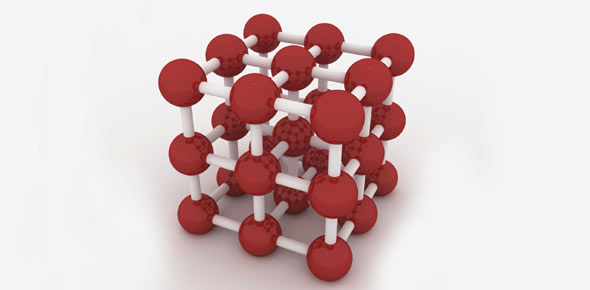# 10 - Science - 10. Chemistry – Atoms And Molecules

6 Questions | Total Attempts: 1584Settings• 1.
From the given examples, form the pair of isotopes.   18 Ar 40, 17 Cl 35, 20 Ca 40, 17 Cl 37
• A.

18 Ar 40, 20 Ca 40

• B.

17 Cl 35, 17 Cl 37

• 2.
From the given examples, form the pair of isobars.   18 Ar 40, 17 Cl 35, 20 Ca 40, 17 Cl 37
• A.

17 Cl 35, 17 Cl 37

• B.

18 Ar 40, 20 Ca 40

• 3.
Molecular mass of nitrogen is 28. Its atomic mass is 14. Find the atomicity of nitrogen.
• A.

28

• B.

14

• C.

2

• D.

3

• 4.
Gram molecular mass of oxygen is 32g. Density of oxygen is 1.429g/cc. Find the gram molecular volume of oxygen.
• A.

1.429g/cc

• B.

32g

• C.

1 lit

• D.

22.4 lit

• 5.
Calculate the gram molecular mass of water from the values of gram atomic mass of hydrogen and of oxygen.
• A.

1

• B.

16

• C.

18

• D.

19

• 6.
One mole of any substance contains 6.023 x 1023 particles. If 3.0115 x 1023  particles are present in CO2. Find the number of moles.
• A.

1

• B.

2

• C.

.05

• D.

0.25

Related TopicsBack to top